# Right triangle + tangent - math problems

#### Number of problems found: 136

• Right triangle trigonometricsCalculate the size of the remaining sides and angles of a right triangle ABC if it is given: b = 10 cm; c = 20 cm; angle alpha = 60° and the angle beta = 30° (use the Pythagorean theorem and functions sine, cosine, tangent, cotangent)
• One side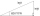One side is 36 long with a 15° incline. What is the height at the end of that side?
• BevelI have bevel in the ratio 1:6. What is the angle and how do I calculate it?
• Sphere in coneA sphere is inscribed in the cone (the intersection of their boundaries consists of a circle and one point). The ratio of the surface of the ball and the contents of the base is 4: 3. A plane passing through the axis of a cone cuts the cone in an isoscele
• ClimbRoad has climbing 1:27. How big is a angle corresponds to this climbing?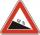Road sign informs the gradient is 10.9%. Calculate the angle which average decreases.
• PilotHow high is the airplane's pilot to see 0.001 of Earth's surface?
• RailwayRailway line had on 5.8 km segment climb 9 permille. How many meters track ascent?The angle of a straight road is approximately 12 degrees. Determine the percentage of this road.
• Hole's angles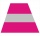I am trying to find an angle. The top of the hole is .625” and the bottom of the hole is .532”. The hole depth is .250” what is the angle of the hole (and what is the formula)?
• Height differenceWhat height difference overcome if we pass road 1 km long with a pitch21 per mille?
• Inscribed and described circle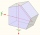Find the radii of a circle inscribed and circumscribed by a regular pentagon whose side measures 3 cm.
• The staircaseThe staircase has a total height of 3.6 m and forms an angle of 26° with the horizontal. Calculate the length of the whole staircase.
• SlopeWhat is the slope of a line with an inclination 6.06 rad?
• TreeHow tall is the tree that observed in the visual angle of 52°? If I stand 5 m from the tree and eyes are two meters above the ground.
• Find the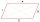Find the content of a regular 12 sided polygon, if its side a = 12 cm.
• SteepleSteeple seen from the road at an angle of 75°. When we zoom out to 25 meters, it is seen at an angle of 20°. What is high?
• n-gon IIWhat is the side length of the regular 5-gon circumscribed circle of radius 11 cm?
• How far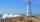From the top of a lighthouse 145 ft above sea level, the angle of depression of a boat 29°. How far is the boat from the lighthouse?On a straight stretch of road is marked 12 percent drop. What angle makes the direction of the road with the horizontal plane?